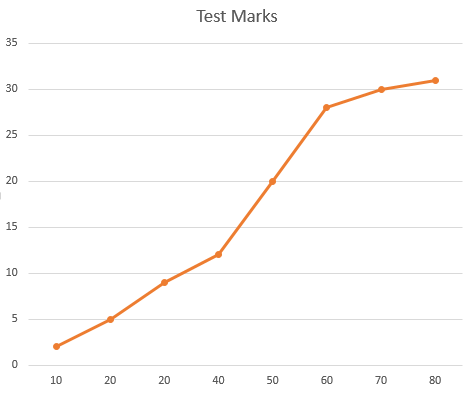Exam-Style Questions.

Problems adapted from questions set for previous Mathematics exams.

1.

GCSE Higher

The following table shows the time taken by fifty people to answer their emails one day.

 Time, t minutes Number of people 0 < t ≤ 10 5 10 < t ≤ 20 12 20 < t ≤ 30 13 30 < t ≤ 40 15 40 < t ≤ 50 5

(a) In which class interval is the median?

(b) How many people took more than 20 minutes?

(c) What is the modal class interval?

(d) Find an estimate for the mean time taken showing all of your working.

2.

GCSE Higher

The following table show data collected by a researcher about the length of monitor lizards living in a park in Bangkok.

Length (cm)Frequency
$$0\lt l \le 50$$9
$$50\lt l \le 100$$13
$$100\lt l \le 150$$22
$$150\lt l \le 200$$19
$$200\lt l \le 250$$11
$$250\lt l \le 300$$3

(a) Which is the modal group?

(b) Calculate an estimate for the mean lizard length.

(c) The researcher claims that the range of lengths must lie between 2m and 3m. Explain how the researcher came to this conclusion.

3.

GCSE Higher

The following table shows the number of times people in a sample group visited the cinema in a six month period.

One of the frequencies is missing.

Cinema Visits Frequency Midpoint
0-4 20 2
5-9 24 7
10-14   12
15-19 7 17

Midpoints are used to work out an estimate for the mean number of visits.

The mean is calculated to be 7.25.

Work out the missing frequency.

4.

GCSE Higher

The table shows the test marks for 31 students.

 Percentage (p) Frequency 5 ≤ p < 15 2 15 ≤ p < 25 3 25 ≤ p < 35 4 35 ≤ p < 45 3 45 ≤ p < 55 8 55 ≤ p < 65 8 65 ≤ p < 75 2 75 ≤ p < 85 1

Marco drew this cumulative frequency graph using the data.Make three criticisms of Marco’s graph.

If you would like space on the right of the question to write out the solution try this Thinning Feature. It will collapse the text into the left half of your screen but large diagrams will remain unchanged.

The exam-style questions appearing on this site are based on those set in previous examinations (or sample assessment papers for future examinations) by the major examination boards. The wording, diagrams and figures used in these questions have been changed from the originals so that students can have fresh, relevant problem solving practice even if they have previously worked through the related exam paper.

The solutions to the questions on this website are only available to those who have a Transum Subscription.

Exam-Style Questions Main Page

Search for exam-style questions containing a particular word or phrase:

To search the entire Transum website use the search box in the grey area below.